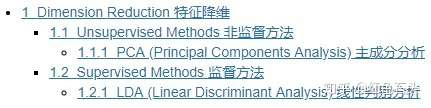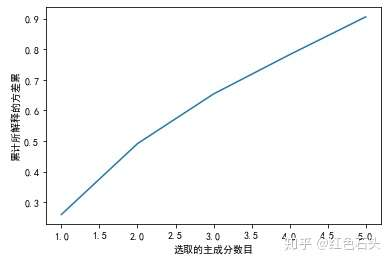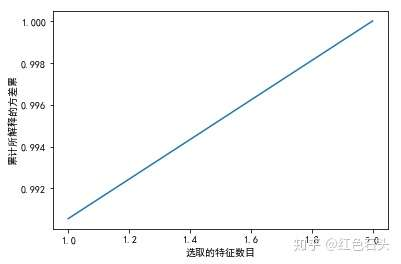# 【完结篇】专栏 | 基于 Jupyter 的特征工程手册：特征降维## 1.1 Unsupervised Methods 非监督方法

### 1.1.1 PCA (Principal Components Analysis) 主成分分析

https://github.com/YC-Coder-Chen/Unsupervised-Notes/blob/master/PCA.md

import numpy as np
import pandas as pd
from sklearn.decomposition import PCA

# 直接载入数据集
from sklearn.datasets import fetch_california_housing
dataset = fetch_california_housing()
X, y = dataset.data, dataset.target # 利用 california_housing 数据集来演示

# 选择前15000个观测点作为训练集
# 剩下的作为测试集
train_set = X[0:15000,:]
test_set = X[15000:,]
train_y = y[0:15000]

# 在使用主成分分析前，我们需要先对变量进行缩放操作，否则PCA将会赋予高尺度的特征过多的权重
from sklearn.preprocessing import StandardScaler
model = StandardScaler()
model.fit(train_set)
standardized_train = model.transform(train_set)
standardized_test = model.transform(test_set)

# 开始压缩特征
compressor = PCA(n_components=0.9)
# 将n_components设置为0.9 =>
# 即要求我们从所有主成分中选取的输出主成分至少能保留原特征中90%的方差
# 我们也可以通过设置n_components参数为整数直接控制输出的变量数目

compressor.fit(standardized_train) # 在训练集上训练
transformed_trainset = compressor.transform(standardized_train) # 转换训练集 (20000,5)
# 即我们从8个主成分中选取了前5个主成分，而这前5个主成分可以保证保留原特征中90%的方差

transformed_testset = compressor.transform(standardized_test) # 转换测试集
assert transformed_trainset.shape == transformed_testset.shape
# 转换后训练集和测试集有相同的特征数

# 可视化 所解释的方差与选取的主成分数目之间的关系

import matplotlib.pyplot as plt
plt.rcParams['font.sans-serif']=['SimHei']
%matplotlib inline

plt.plot(np.array(range(len(compressor.explained_variance_ratio_))) + 1,
np.cumsum(compressor.explained_variance_ratio_))
plt.xlabel('选取的主成分数目')
plt.ylabel('累计所解释的方差累')
plt.show(); # 前5个主成分可以保证保留原特征中90%的方差## 1.2 Supervised Methods 监督方法

### 1.2.1 LDA (Linear Discriminant Analysis) 线性判别分析

import numpy as np
import pandas as pd
from sklearn.discriminant_analysis import LinearDiscriminantAnalysis as LDA

# LDA仅适用于分类问题
# 载入数据集
X, y = iris.data, iris.target

# iris 数据集使用前需要被打乱顺序
np.random.seed(1234)
idx = np.random.permutation(len(X))
X = X[idx]
y = y[idx]

# 选择前100个观测点作为训练集
# 剩下的50个观测点测试集

train_set = X[0:100,:]
test_set = X[100:,]
train_y = y[0:100]
test_y = y[100:,]

# 在使用主成分分析前，我们需要先对变量进行缩放操作
# 因为LDA假定数据服从正态分布

from sklearn.preprocessing import StandardScaler # 我们也可以采用幂次变换
model = StandardScaler()
model.fit(train_set)
standardized_train = model.transform(train_set)
standardized_test = model.transform(test_set)

# 开始压缩特征
compressor = LDA(n_components=2) # 将n_components设置为2
# n_components <= min(n_classes - 1, n_features)

compressor.fit(standardized_train, train_y)  # 在训练集上训练
transformed_trainset = compressor.transform(standardized_train) # 转换训练集 (20000,2)
transformed_testset = compressor.transform(standardized_test) # 转换测试集
assert transformed_trainset.shape == transformed_testset.shape
# 转换后训练集和测试集有相同的特征数

# 可视化 所解释的方差与选取的特征数目之间的关系
import matplotlib.pyplot as plt
plt.plot(np.array(range(len(compressor.explained_variance_ratio_))) + 1,
np.cumsum(compressor.explained_variance_ratio_))
plt.xlabel('选取的特征数目')
plt.ylabel('累计所解释的方差累')
plt.show(); # LDA将原始的4个变量压缩为2个，这2个变量即能解释100%的方差### 觉得文章有用就打赏一下文章作者

#### 支付宝扫一扫打赏#### 微信扫一扫打赏